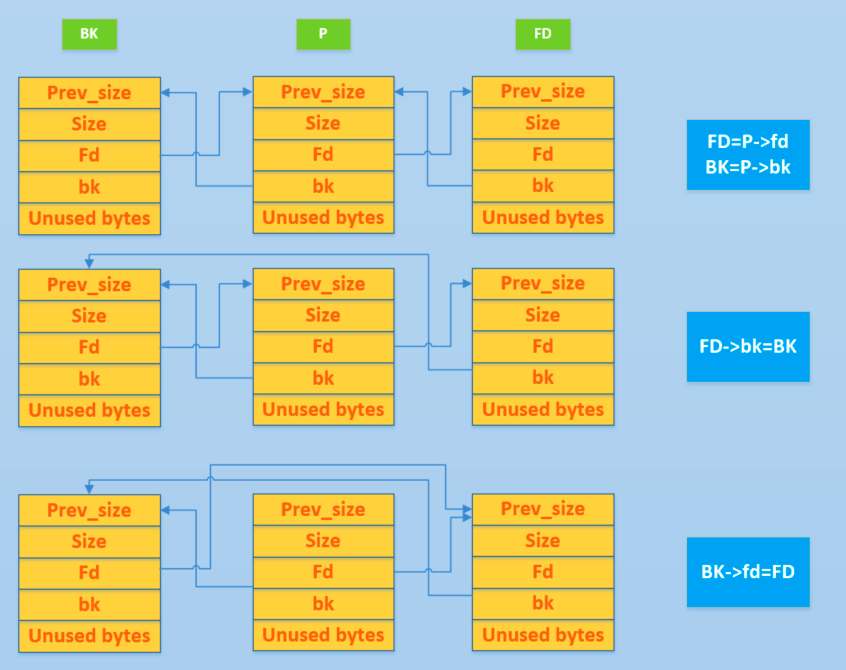# 基本操作¶

Unlink is used to take out an element in a doubly linked list (only free chunks), which may be used in the following places.

• malloc

• Get chunks from a large bin of exactly the right size.

• It should be noted here that fastbin and small bin do not use unlink, which is why vulnerabilities often appear here.
• Unlink is also not used when traversing the unsorted bin in turn.
• Take a chunk from the bin larger than the bin where the requested chunk is located.
• free

• Forward merge, merge physical neighbor high address free chunks (except top chunk).
• malloc_consolidate

• Forward merge, merge physical neighbor high address free chunks (except top chunk).
• realloc

• Forward expansion, merging physical adjacent high address free chunks (except top chunk).

Since unlink is used very frequently, unlink is implemented as a macro, as follows

/* Take a chunk off a bin list */

#define unlink (AV, P, BK, FD) {
// Since P is already in the doubly linked list, there are two places to record its size, so check if the size is the same.
if (__builtin_expect (chunksize(P) != prev_size (next_chunk(P)), 0))      \

malloc_printerr ("corrupted size vs. prev_size");               \

FD = P->fd;                                                                      \

BK = P->bk;                                                                      \

/ / Prevent the attacker from simply tampering with the fd and bk of the free chunk to achieve arbitrary write effects.
if (__builtin_expect (FD->bk != P || BK->fd != P, 0))                      \

malloc_printerr (check_action, "corrupted double-linked list", P, AV);  \

else {                                                                      \

FD->bk = BK; \
BK->fd = FD; \
/ / The following mainly consider the modification of the nextsize doubly linked list corresponding to P

// If P->fd_nextsize is NULL, it means that P is not inserted into the nextsize list.
// Then there is no need to modify the nextsize field.
// There is no way to determine the bk_nextsize field, which may cause problems.
&& __builtin_expect (P->fd_nextsize != NULL, 0)) {                      \

/ / Similar to the small chunk check idea
if (__builtin_expect (P->fd_nextsize->bk_nextsize != P, 0)              \

|| __builtin_expect (P->bk_nextsize->fd_nextsize != P, 0))    \

malloc_printerr (check_action,                                      \

"corrupted double-linked list (not small)",    \

P, AV); \
// This shows that P is already in the nextsize list.
// If the FD is not in the nextsize list
if (FD->fd_nextsize == NULL) {                                      \

// If the double-linked list of nextsize is only P itself, then take P directly
// Let FD be a string of nextsize
if (P->fd_nextsize == P)                                      \

FD->fd_nextsize = FD->bk_nextsize = FD; \
else {                                                              \

// Otherwise we need to insert the FD into the double-linked list formed by nextsize
FD->fd_nextsize = P->fd_nextsize;                              \

FD->bk_nextsize = P->bk_nextsize;                              \

P->fd_nextsize->bk_nextsize = FD;                              \

P->bk_nextsize->fd_nextsize = FD;                              \

}                                                              \

} else {                                                              \

// If you are, take it straight away.
P->fd_nextsize->bk_nextsize = P->bk_nextsize;                      \

P->bk_nextsize->fd_nextsize = P->fd_nextsize;                      \

}                                                                      \

}                                                                      \

}                                                                              \

}


Here we introduce the unlink of small bin as an example. For unbin of large bin, it is similar to just one additional size.It can be seen that the final fd and bk pointers of P have not changed, but when we go through the entire doubly linked list, we have not traversed the corresponding linked list. This is not useful for change, because we can sometimes use this method to leak addresses.

• P is located in the head of the doubly linked list, bk leaks
• P is located at the end of the doubly linked list, fd leaks
• When the doubly linked list contains only one free chunk, P is in the doubly linked list, and both fd and bk can leak.
• P is located in the head of the doubly linked list, fd leaks
• P is in the doubly linked list, both fd and bk can leak
• P is located at the end of the doubly linked list, bk leaks

note

• The header here refers to the chunk pointed to by the fd of bin, which is the latest chunk added in the doubly linked list.
• The tail here refers to the chunk pointed to by bk of bin, which is the first chunk added in the doubly linked list.

At the same time, for both fd, bk and fd_nextsize, bk_nextsize, the program will check if fd and bk meet the corresponding requirements.

// fd bk
if (__builtin_expect (FD->bk != P || BK->fd != P, 0))                      \

malloc_printerr (check_action, "corrupted double-linked list", P, AV);  \

// next_size related

if (__builtin_expect (P->fd_nextsize->bk_nextsize != P, 0)              \

|| __builtin_expect (P->bk_nextsize->fd_nextsize != P, 0))    \

malloc_printerr (check_action,                                      \

"corrupted double-linked list (not small)",    \

P, AV);


It seems to be normal. Let us take fd and bk as an example. The bk of the forward chunk of P is naturally P, and the fd of the backward chunk of P is also naturally P. If we do not check the corresponding, we can modify the fd and bk of P, so that the effect of writing at any address can be easily achieved. For a more detailed example, see the Unlink section of the Utilization section.

Note: The prev_inuse bit recorded by the first chunk of the heap defaults to 1.

## malloc_printerr¶

The malloc_printerr function is called when an error is detected in glibc malloc.

static void malloc_printerr(const char *str) {

__libc_message(do_abort, "%s\n", str);

__builtin_unreachable();

}


Mainly will call __libc_message to execute the abort function, as follows

  if ((action & do_abort)) {
if ((action & do_backtrace))

BEFORE_ABORT(do_abort, written, fd);

/* Kill the application.  */

abortion();
}


In the abort function, flibsh stream will be used when glibc is still 2.23.

  /* Flush all streams.  We cannot close them now because the user

might have registered a handler for SIGABRT.  */

if (stage == 1)

{

++stage;

fflush (NULL);

}